# Angular momentum coupling

In quantum mechanics, angular momentum coupling is the procedure of constructing eigenvectors of a system's angular momentum out of angular momentum eigenvectors of its subsystems. The historic example of a system to which angular momentum coupling is applied, is an atom with N > 1 electrons (the subsystems of the atom). Each electron has its own orbital angular momentum, i.e., is in an eigenstate of its own angular momentum operator. Angular momentum coupling is the construction of an N-electron eigenstate of the total atomic angular momentum operator out of the N individual electronic angular momentum eigenstates.

Other examples are the coupling of spin- and orbital-angular momentum of an electron (where we see the spin and the orbital motion as subsystems of a single electron) and the coupling of nucleonic spins in the shell model of the nucleus.

## Application

The coupling of angular momenta of subsystems to total angular momentum is useful and applicable when two conditions are satisfied.

1. In the absence of interactions between the subsystems the angular momenta of the subsystems are constants of the motion. That is, when the interactions between the subsystems are switched off, each individual angular momentum commutes with the Hamiltonian. Many physical theories have this zeroth order approximation as point of departure.
2. When next the subsystems are assumed to be interacting (are exerting forces on each other), then in general the individual angular momenta cease to be constants of the motion. However, if their sum, the total angular momentum, is a constant of the motion even after the interactions have been switched on, angular momentum coupling will be useful in solving the Schrödinger equation of the total system.

These two conditions are surprisingly often fulfilled due to the fact that they almost always follow from rotational symmetry—the symmetry of spherical systems and isotropic interactions. The angular momentum is a constant of the motion, in either of two situations: (i) The system is spherical symmetric, or (ii) the system moves (in quantum mechanical sense) in isotropic space. It can be shown that in both cases the total angular momentum operator of the system commutes with its Hamiltonian. By Heisenberg's uncertainty relation this means that the angular momentum of the system can assume a sharp (i.e., eigen-) value simultaneously with the energy (eigenvalue of the Hamiltonian) of the system.

The standard example of a spherical symmetric system is an atom, while a (rigid) molecule moving in a field-free space is an example of the second kind of system. A rigid (non-vibrating) molecule can be seen as a rigid rotor, which moving in field-free space, has a conserved angular momentum. This observation lies at the basis of microwave spectroscopy.

### Example: two-electron atom

As an example of angular momentum coupling in a spherical symmetric system, we consider a two-electron atom.

First, assume that there is no electron-electron interaction (or other interactions such as spin-orbit coupling), but that only the electron-nucleus Coulomb attraction is operative. In this simplified model the atomic Hamiltonian is a sum of kinetic energies of the two electrons and the spherical symmetric electron-nucleus interaction. The kinetic energy of the nucleus (or, more precisely of the center of mass of the atom), which is three to four orders of magnitude smaller than the kinetic energy of the electrons, is neglected.

The orbital angular momentum l(i) (a vector operator) of electron i (with i = 1 or 2) commutes with the total Hamiltonian. Both operators, l(1) and l(2), are constant of the motion. It can be shown that the commutation of l(i) with the simplified Hamiltonian has the consequence that electron i can be rotated around the nucleus independently of the other electron; upon rotation nothing happens to the energy of either electron (which is easy to understand because the electron-electron interaction is off and the nucleus is spherical symmetric).

Switching on the electron-electron interaction, which depends on the distance d(1,2) between the electrons, we get the more exact Hamiltonian H. Clearly, only a simultaneous and equal rotation of the two electrons will leave d(1,2) invariant. Independent rotation of only one electron will change the distance d(1,2) to the other electron and hence the electron-electron interaction energy.

It can be shown that this change in distance implies that neither l(1) nor l(2) commute separately with H, but their sum L = l(1) + l(2) still does. The operator L commutes with H if and only if a simultaneous rotation of the two electrons leaves H invariant.

Given eigenstates of l(1) and l(2), the construction of eigenstates of L from them is the coupling of the angular momenta of electron 1 and 2. It is fairly easy to construct eigenstates of L by the use of the explicit form of Clebsch-Gordan coefficients. The coupled states are labeled by a non-negative integer L. It can be shown that eigenstates labeled by different L do not mix under the total Hamiltonian H (the one including electron-electron interaction), which means that eigenvectors of H are completely contained in a space of a single definite L. This fact is a great aid in obtaining the eigenvectors of H, i.e., in the solution of the time-independent Schrödinger equation of the example two-electron atom.

## Group theoretical background

A spherical system has symmetry group SO(3), the special orthogonal group in three dimensions. This group consists of orthogonal 3 × 3 matrices with unit determinant. The group is closely related to the spin rotation group SU(2) (special unitary group), which consists of unitary 2 × 2 matrices with unit determinant. Both groups are Lie groups and have isomorphic Lie algebras. The Lie algebras are 3-dimensional and are generated by the components of orbital-angular momentum through the commutation relations$[l_x, l_y] = i l_z \,$

and cyclic permutation of x, y, and z (we put$\hbar = 1$).

To simplify the discussion we restrict the attention to SO(3), the extension to SU(2) is not difficult. As is usual, one goes from the Lie algebra to the group by exponentiation: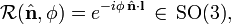$\mathcal{R}(\hat{\mathbf{n}}, \phi) = e^{-i \phi\, \hat{\mathbf{n}}\cdot \mathbf{l}} \, \in\, \mathrm{SO(3)},$

where${\mathcal{R}(\hat{\mathbf{n}}, \phi)}$ represents a rotation around the unit vector${\hat{\mathbf{n}} }$ over an angle φ and l ≡ (lx, ly, lz). In the two-electron atom example above, we met l as the orbital angular momentum of an electron.

By expanding the exponential operator it follows that the components of l commute with the Hamiltonian if and only if the rotation operator${\mathcal{R}(\hat{\mathbf{n}}, \phi)}$ commutes with the Hamiltonian,$[H, l_x] = [H, l_y] = [H, l_z] = 0 \Longleftrightarrow [H, \mathcal{R}(\hat{\mathbf{n}}, \phi)] = 0, \quad \forall\, \hat{\mathbf{n}}\;\;\hbox{and}\;\; \forall \phi.$

This relation shows very succinctly the correspondence between rotational symmetry and angular momentum.

If the electron-electron interaction is switched off in the two-electron atom, the symmetry group of the atom is the outer product group SO(3) × SO(3). If the interaction is switched on, the symmetry of the atom is lowered to the inner product group, which is isomorphic to SO(3) and is the subgroup of SO(3) × SO(3) consisting of simultaneous and equal rotations of the two electrons.

The inner product group consists of the following elements$e^{-i \phi\, \hat{\mathbf{n}}\cdot \mathbf{l}} \otimes e^{-i \phi\, \hat{\mathbf{n}}\cdot \mathbf{l}}= e^{-i \phi\, \hat{\mathbf{n}}\cdot (\mathbf{l}\otimes 1 + 1\otimes \mathbf{l}) } = e^{-i \phi\, \hat{\mathbf{n}}\cdot \mathbf{L}},$

where we introduced the total angular momentum operator,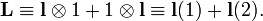$\mathbf{L} \equiv \mathbf{l}\otimes 1 + 1\otimes \mathbf{l} \equiv \mathbf{l}(1)+ \mathbf{l}(2).$

We see here clearly exhibited the correspondence between the total two-electron operator L and the simultaneous rotation of the two electrons around the same axis n over the same angle φ.

Construction of eigenstates of L2 = Lx2 + Ly2 + Lz2 out of eigenstates of l(1)2 and l(2)2, i.e., angular momentum coupling, is equivalent to the group theoretical subduction of the outer direct product to the inner direct product,$\mathrm{SO(3)} \times \mathrm{SO(3)} \downarrow \mathrm{SO(3)}.$

Subduction implies in this case the reduction of a reducible tensor product representation to (a direct sum of) irreducible representions of SO(3).

##  Triangular conditions

In order to indicate that the theory holds for any kind of angular momentum, we will write j instead of l, because the latter symbol is often reserved for orbital angular momentum. If subsystem 1 has an angular momentum operator j(1) and subsystem 2 has an angular momentum operator j(2), the total system, consisting of these two subsystems, has an angular momentum operator Jj(1) + j(2). The components of the latter operator can also be written as Jkjk ⊗1 + 1⊗jk . If subsystem 1 is in an eigenstate of j2(1) with quantum number j1 and if subsystem 2 is in an eigenstate of j2(2) with quantum number j2 then the allowed eigenstates of J2 are those with quantum number J satisfying the triangular conditions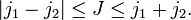$|j_1-j_2| \le J \le j_1+j_2.$

A slightly different formulation is the following. The statement that quantum system 1 is in an eigenstate of j(1) with quantum number j1 means that the state belongs to a 2j1 + 1 dimensional linear space$V_{j_1}$, an eigenspace of j2(1) with eigenvalue j1(j1 + 1), see this article for a discussion of this. Likewise the angular momentum eigenstate of quantum system 2 belongs to a 2j2 + 1 dimensional linear space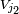$V_{j_2}$, an eigenspace of j2(2) with eigenvalue j2(j2 + 1). The triangular conditions state that the tensor product space decomposes as the following orthogonal direct sum of eigenspaces of J2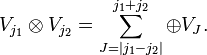$V_{j_1}\otimes V_{j_2} = \sum_{J=|j_1-j_2|}^{j_1+j_2} \oplus V_J.$

Note the limits on the summation and note also that an eigenspace$V_{J}$ of certain quantum number J occurs only once (provided J is within the limits, otherwise it does not occur), in other words the multiplicity of J in the tensor product space with fixed j1 and j2 is zero or one. This non-trivial result is sometimes referred to as the Clebsch-Gordan theorem after the two German nineteenth century mathematicians who discovered it (in the context of invariant theory).

The result also has a group theoretical implication. The irreducible representations (irreps) of the group SO(3) are labelled by j. As we discussed above, the outer direct product of two irreps of SO(3), one labeled by j1 and one labeled by j2, decomposes into a direct sum of irreps of the inner direct product group. The latter irreps are labeled by J and this label satisfies the triangular conditions. Of course, this result is no coincidence, but a consequence of the fact the components of the angular momentum operator span the Lie algebra of SO(3).

###  Proof of the triangular conditions

Physicists are so familiar with the triangular conditions that many of them do not realize (or have forgotten) that the proof of the conditions is non-trivial, at least from the angular momentum (Lie algebra) point of view. From the point of view of the global groups SO(3) or SU(2) the result is proved in four lines by means of character theory, see Ref. Because the latter, short, proof requires some group theoretical knowledge, we now present the standard "bookkeeping" type of proof, that, although fairly complicated, requires no new knowledge.

We will decompose the (2j1+1)(2j2+1)-dimensional tensor product space into eigenspaces of J and Jz for fixed j1 and j2.

The first important observation is that a simple tensor product of eigenvectors is an eigenvector of Jzjz ⊗1 + 1⊗jz,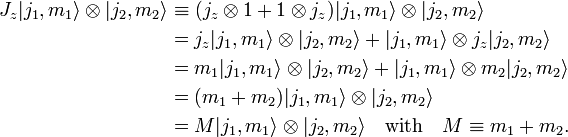\begin{align} J_z |j_1,m_1\rangle \otimes|j_2,m_2\rangle& \equiv (j_z\otimes 1 + 1\otimes j_z) |j_1,m_1\rangle \otimes|j_2,m_2\rangle\\ &= j_z|j_1,m_1\rangle \otimes |j_2,m_2\rangle + |j_1,m_1\rangle \otimes j_z |j_2,m_2\rangle \\ &= m_1|j_1,m_1\rangle \otimes |j_2,m_2\rangle + |j_1,m_1\rangle \otimes m_2|j_2,m_2\rangle \\ &= (m_1+m_2) |j_1,m_1\rangle \otimes |j_2,m_2\rangle \\ &=M|j_1,m_1\rangle \otimes |j_2,m_2\rangle \quad \hbox{with}\quad M\equiv m_1+m_2. \end{align}

The second observation needed in the proof is the following. Since J is an angular momentum operator, its eigenspaces have the general structure discussed in the article on the quantum angular momentum operator. Specifically, the space possesses a vector of maximum eigenvalue of Jz with M = J. This vector is the top of a ladder of 2J+1 states of decreasing M (by steps of −1) with the bottom of the ladder being the state with M = −J. Since the tensor product space is invariant (stable) under the components of J, it contains only full (2J+1)-dimensional eigenspaces ("complete ladders") of J2. If we have found one element of a ladder (a simultaneous eigenvector of Jz and J2), it follows that the other elements of the ladder are also present in the tensor product space.

As enumeration ("bookkeeping") types of proof are easier by way of example, we consider the case j2 = 2 and j1 = 3 together with the (5 × 7)-dimensional tensor product space depicted in the figure below. Consider the central diagram at the top of the figure. Evidently, in this diagram there is only one product vector ("green dot") with M = 5. This dot stands for the product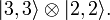$|3,3\rangle\otimes |2,2\rangle .$

According to what is just said, this product must be the top of a 2×5 + 1 = 11 dimensional ladder of eigenvectors with J = 5 and M varying from 5 to −5 in steps of −1. So we know that the 11-dimensional eigenspace of J2 with J = 5 appears once in the angular momentum coupling. At a glance we see that no higher M—and hence no higher J—appears.

Consider next the diagonal marked by M = 4, still in the topmost diagram. The two green dots with this M stand for the two mutually orthogonal—and hence linearly independent—product vectors:$|3,3\rangle\otimes |2,1\rangle \quad \hbox{and}\quad |3,2\rangle\otimes |2,2\rangle.$

The third important observation, slightly complicating the proof, is that neither of the two product vectors are eigenvectors of J2. However, two linear combinations of the products are eigenvectors of J2, both with M = 4. Since we have found already that there must be an M = 4 eigenvector of Jz with J = 5, one linear combination is already accounted for, and we can take it out of this space. The single remaining linear combination will belong to another eigenspace of J2.Angular momentum coupling of j1=3 and j2=2. Green dots are product vectors. The endings of the diagonal lines in the diagrams are marked (on the right hand sides and the bottoms) by M = m1 + m2. See the text for the explanation of the red hooks.

We can repeat this argument for the three products with M = 3. One linear combination of the three is already accounted for and can be removed, two linear combinations remain. Going down all the way to M = −5, we see that we can remove the hook ("gnomon") representing 11 eigenfunctions of J2 with J = 5.

After removal of the outer red hook we obtain the leftmost diagram in the second row. As we just discussed there is only one green dot with M = 4. (Strictly speaking the dot does no longer stand for a product, but rather for a single linear combination of products of fixed M). Again there must be whole ladder below it: the hook marked by J = 4. We take this hook out, remembering that we found J = 4, and continue this way, until we end up with three green dots with highest M = 1: this stands for the eigenspace with J = 1.

Summarizing:

J = 5, 4, 3, 2, 1

with every J value appearing once. This corresponds in this example (where j1≥j2) to:

J = j1+j2, j1+j2−1, ..., j1−j2.

After the special case, the general case will be clear. See the figure on the right for the case j1 ≥ j2. Red hooks can be peeled off with unique quantum numbers running from J = j1+j2 to j1−j2+1. Finally a straight line with J = j1−j2 remains, which represents the final eigenspace of J2.

Note finally that the case j2 > j1 is obtained simply by interchanging 1 and 2 in all of the above. The fact that J ≥ 0, is consistent with taking the absolute value of the the difference of quantum numbers in the lower limit.

It is of some interest to check the dimension of the tensor product space, before and after direct sum decomposition. Before expansion it is, as we saw, simply (2j1+1)(2j2+1). After expansion it is (for j1 ≥ j2):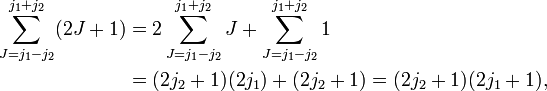\begin{align} \sum_{J=j_1-j_2}^{j_1+j_2}(2J+1) &= 2\sum_{J=j_1-j_2}^{j_1+j_2}J + \sum_{J=j_1-j_2}^{j_1+j_2}1 \\ &= (2j_2+1)(2j_1) + (2j_2+1) = (2j_2+1)(2j_1+1), \end{align}

where we used the sum of the arithmetic series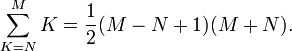$\sum_{K=N}^{M} K = \frac{1}{2}(M-N+1)(M+N).$

## Note and reference

1. A constant of the motion is also referred to as a conserved property. It is a time-independent observable that commutes with the Hamiltonian of the system.
2. The name derives from the corresponding conditions on the sides of a triangle. If a, b, and c are the lengths of the sides of a triangle, then |a-c| ≤ ba+c.
3. J. D. Talman, Special Functions, A Group Theoretic Approach, (based on lectures by E.P. Wigner), W. A. Benjamin, New York (1968).

• Edmonds, A. R. (1957). Angular Momentum in Quantum Mechanics. Princeton, New Jersey: Princeton University Press. ISBN 0-691-07912-9.
• Messiah, Albert (1981). Quantum Mechanics (Volume II), 12th edition. New York: North Holland Publishing. ISBN 0-7204-0045-7.
• Biedenharn, L. C.; Louck, J. D. (1981). “Chapter 3.11”, Angular Momentum in Quantum Physics. Reading: Addison-Wesley. ISBN 0-201-13507-8.
• Cohen-Tannoudji, C.; Diu, B. and Laloë, F. (1977). “Chapter X”, Quantum Mechanics (Volume II). New York: John Wiley. ISBN 0-471-16434-8.
• Brink, D. M.; Satchler, G. R. (1993). “Chapter 2”, Angular Momentum, 3rd edition. Oxford: Clarendon Press. ISBN 0-19-851759-9.